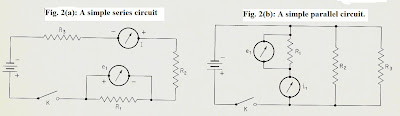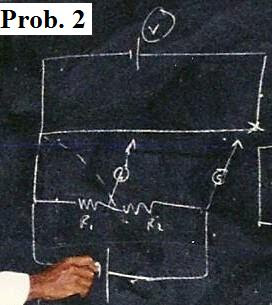## Wednesday, June 29, 2011

### Introducing Basic Physics (Series & Parallel Circuits)- Pt. 22We now look at series and parallel circuits using the Wheatstone Bridge method. The same set up is used as shown in the photo for Part 21 and the circuit diagram (for the slide wire set up) is in Fig. 1. The separate circuit diagrams are shown in the accompanying diagrams (Fig. 2a and 2b)for the series and parallel cases.

Apparatus used:

i) Wheatstone Bridge

ii) d.c. voltmeter (0- 3 V)

iii) ammeter (0- 1A, 0- 10A)

iv) sensitive galvanometer

v) low voltage d.c. source (2V or under)

vi) wires for connections

Main Background points:

1. Series Circuits.

The general diagram is such as shown in Fig. 2(a). Here, separate components are connected in such a way the source of emf (battery) forces electric current through all components (e.g. resistors) in sequence. Once steady conditions are established the same current flows through all resistances. The total resistance is the sum of the individuals:

R = R1 + R2 + R3 + ........R_n

where R1, R2 are the resistances of the separate resistors.

The voltage in a series circuit is such that each component causes a drop in potential and the sum of all potential drops is equal to the emf applied to the total circuit:

E = V1 + V2 + V3 + ......V_n

Current in a series circuit is constant so the total is the same flowing through any given component:

I = I1 = I2 = I3 etc.

2. Parallel circuits

This set-up is such as shown in Fig. 2(b). In parallel circuits the current paths branch, hence are not sequential. Since several paths are available for current flow instead of just one, then the total resistance is less than the resistance of any ONE of the alternative paths, or resistors. The total is:

1/R = 1/R1 + 1/R2 + 1/ R3 + .....1/R_n

Thus, the reciprocal of the total resistance of a parallel circuit is just the sum of the reciprocals of the separate resistances.

Special case:

This is for two in parallel, which makes easy computation for the total:

E.g.

1/R = 1/R1 + 1/R2 = R1 + R2 / (R1 R2)

Then: R = (R1 R2)/ (R1 + R2)

The voltage in a parallel circuit is the same across any component of the circuit, as it is across the circuit as a whole, so:

E = V1 = V2 = V3 etc.

The current in a parallel circuit is the sum of all the currents flowing in the separate components, i.e.

I = I1 + I2 + I3..+

Procedures for experiment

A) Series circuit.

Use the Wheatstone Briddge slide wire set up (Fig. 1) to determine the resistance of each resistor and use an emf no larger than 1.0 V. Connect all resistors in series and first measure the total resistance with Wheatstone Bridge (R_T). Connect ammeter and voltmeter across each as indicated (Fig. 2(a)) to obtain resistance based on Ohm's law, R= V/I)

B) Parallel circuit.

Use the same Wheatstone Bridge, but now connecting all the resistors in parallel using the resistance board (see, e.g. the left side of the photograph in Part 21 of the set up). Use the voltmeter and ammeter to take all necessary readings as per Part (A) but now with Rs in parallel. Take care in selection of meter readings so accurate readings will be obtained (preferably to three signficant figures).

Practical Problem:

A student at Harrison College uses the Wheatstone Bridge method to connect up resistors in parallel analogous to Fig. 2(b) He uses his ammeter and voltmeter (0- 1V) to obtain the following readings.

For R1: I1 = 0.50 A, V1 = 0.75 V

For R2: I2 = 0.25 A, V2 = 0.75 V

For R3: I3 = 0.75 A, V2 = 0.75V

a) Find the individual resistances based on his measurements.

b) Find the total resistance.

Solution:

a) Using Ohm's law, one obtains:

R1 = V1/ I1 = 0.75V/ 0.50A = 1.50 ohms

R2 = V2/ I2 = 0.75V/0.25A = 3.0 ohms

R3 = V3/I3 = 0.75V/ 0.75A = 1.0 ohms

b) The total for resistors in parallel:

1/R = 1/ R1 + 1/R2 + 1/ R3

1/R = 1/ 1.5 + 1/3 + 1/1

1/R = 1/(3/2) + 1/3 + 1 = 2/3 + 1/3 + 1 = 2

So: R = 1/2 = 0.5 ohms

Check: Is this smaller than any of the individual values? Yes!

Other Problems:

1)Two resistances of 20 ohms and 5 ohms are connected in parallel by a student. He then connects this combination in series with a 3 ohm resistance and a battery of 1 ohm resistance.

a) Draw a conventional diagram of the circuit (i.e. not in Wheatstone Bridge format).

b) Find the resistance of the resistors in parallel.

c) Find the total resistance of the circuit.

2) The photo shows a sketch of a Wheatstone Bridge Circuit at Harrison College, to be used to find the resistances of two resistors (R1 and R2) connected as shown, with two differing positions of the galvanometer. The total resistance R is also to be taken.

A student using the diagram finds his voltmeter reads 1.0 V and the total resistance is 4 ohms when the slide wire is at the end position noted.

If the balance for obtaining G = 0 (e.g. galvanometer reading zero) is the intermediate position, and the slide wire is then at 45 cm, find the values of R1 and R2.

3) A resistance R2 is connected in parallel with a resistance R1. What resistance R3 must be connected in series with the combination of R1 and R2 so that the equivalent resistance is equal to the resistance R1? Draw a circuit diagram of the arrangement.

4) Three equal resistors are connected in series. When a certain potential difference is applied across the combination, the total power dissipated is 10 watts. (Note: Power = V x I, voltage x current).

What power would be dissipated if the three resistors were connected in parallel across the same potential difference?

#### 1 comment:

Caleb Shay said...

I just want to thank you again for these physics blogs! I have been following them from the start and enjoy the experiments as well as the problems. The electricity section is one of my faves, and I hope you also plan to do some stuff on nuclear energy, radioactivity and relativity!